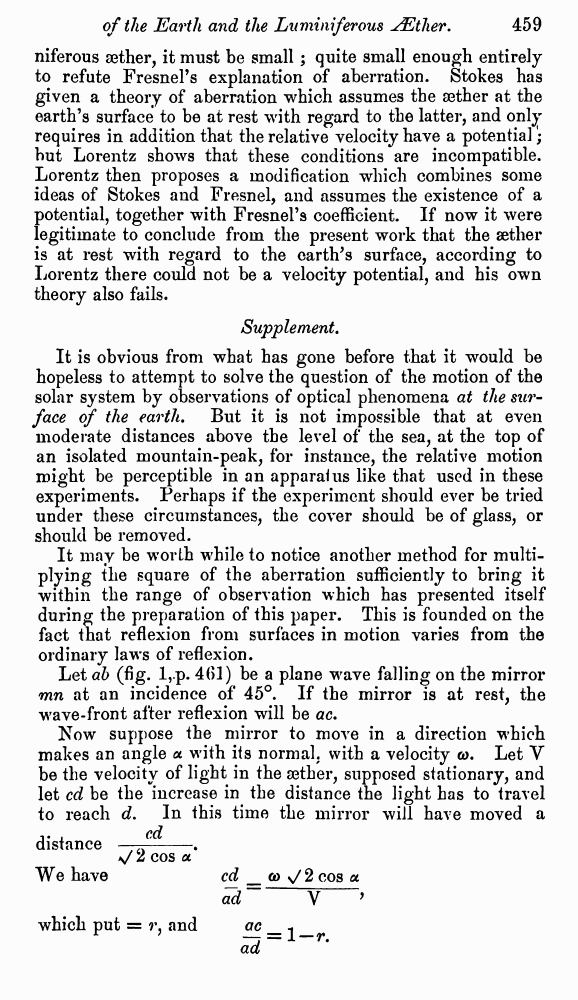# A. Michelson and E. Morley. On the Relative Motion of the Earth and the Luminiferous Æther. // Phil. Mag. S. 5. Vol. 24. No. 151. Dec. 1887.

В начало   Другие форматы   <<<     Страница 458   >>>449  450  451  452  453  454  455  456  457  458 459  460  461  462  463  464  465  466 The results of the observations are expressed graphically in fig. 6. The upper is the curve for the observations at noon, and the lower that for the evening observations. The dotted curves represent one eighth of the theoretical displacements. It seems fair to conclude from the figure that if there is any Fig. 6. displacement due to the relative motion of the earth and the luminiferous æther, this cannot be much greater than 0.01 of the distance between the fringes. Considering the motion of the earth in its orbit only, this displacement should be The distance D was about eleven metres, or 2 × 107 wavelengths of yellow light ; hence the displacement to be expected was 0.4 fringe. The actual displacement was certainly less than the twentieth part of this, and probably less than the fortieth part. But since the displacement is proportional to the square of the velocity, the relative velocity of the earth and the æther is probably less than one sixth the earth’s orbital velocity, and certainly less than one fourth. In what precedes, only the orbital motion of the earth is considered. If this is combined with the motion of the solar system, concerning which but little is known with certainty, the result would have to be modified ; and it is just possible that the resultant velocity at the time of the observations was small, though the chances are much against it. The experiment will therefore be repeated at intervals of three months, and thus all uncertainty will be avoided. It appears from all that precedes reasonably certain that if there be any relative motion between the earth and the lumi- niferous æther, it must be small ; quite small enough entirely to refute Fresnel’s explanation of aberration. Stokes has given a theory of aberration which assumes the æther at the earth’s surface to be at rest with regard to the latter, and only requires in addition that the relative velocity have a potential; but Lorentz shows that these conditions are incompatible. Lorentz then proposes a modification which combines some ideas of Stokes and Fresnel, and assumes the existence of a potential, together with Fresnel’s coefficient. If now it were legitimate to conclude from the present work that the æther is at rest with regard to the earth's surface, according to Lorentz there could not be a velocity potential, and his own theory also fails. Supplement. It is obvious from what has gone before that it would be hopeless to attempt to solve the question of the motion of the solar system by observations of optical phenomena at the surface of the earth. But it is not impossible that at even moderate distances above the level of the sea, at the top of an isolated mountain-peak, for instance, the relative motion might be perceptible in an apparatus like that used in these experiments. Perhaps if the experiment should ever be tried under these circumstances, the cover should be of glass, or should be removed. It may be worth while to notice another method for multiplying the square of the aberration sufficiently to bring it within the range of observation which has presented itself during the preparation of this paper. This is founded on the fact that reflexion from surfaces in motion varies from the ordinary laws of reflexion. Let ab (fig. l, p. 461) be a plane wave falling on the mirror mn at an incidence of 45°. If the mirror is at rest, the wave-front after reflexion will be ac. Now suppose the mirror to move in a direction which makes an angle α with its normal, with a velocity ω. Let Y be the velocity of light in the æther, supposed stationary, and let cd be the increase in the distance the light has to travel to reach d. In this time the mirror will have moved a distance We have which put = r, and